## Saturday, February 8, 2020

### Quantum Oceanography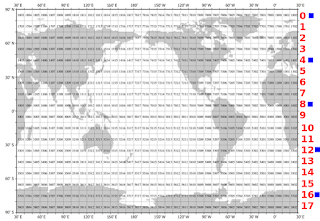Fig. 1 WOD Layer Concept
I. A Thing Or Two

One thing is certain.

When the explorers of the deeps get way down to the Midnight Zone, they will find quanta (Midnight Zone).

They will find photons, the quanta of electromagnetic radiation.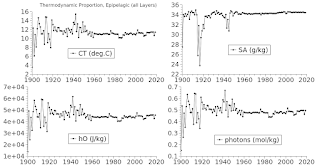Fig. 2 Standard TEOS-10

Some of the photons in seawater are 'light' (the visible spectrum), some are ultra-invisible (ultraviolet above the visible spectrum), and some are infra-invisible (infrared below the visible spectrum).

The invisible spectrum photons have things in common, one of which is that they are not sufficiently studied by oceanographers.

On the other hand all photons have something in common, which is e=hv (the amount of energy in them is calculated the same way).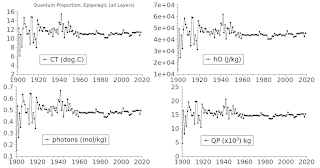Fig. 3 Standard Quantum Proportion

But most of them in the ocean are essentially ghosts, i.e. they are not visible to researchers (The Ghost Photons, 2, 3).

They are not visible because they are not in the visible spectrum ('light'), no, they are in the infrared spectrum which the human eye can't see ("out of sight out of mind"?).

So many of the papers I read seem to have forgotten that visible light in the ocean is not the only game in town.

The focus in so many papers is mostly about what ocean plants and animals do with visible photons, not what the seawater itself does with invisible photons (e.g. here, here, here, here).

Are we forgetting that "the equivalent of between three and six atomic bombs per second " goes into the oceans (As Our World Burns)?

Forgetting that most 'ocean heat' is composed of invisible photons in the infrared range of electromagnetic energy is actually forgetting most of the photons in the oceans.

But, thanks to Planck, Einstein, and Gibbs (boosting Dredd Blog 🙏) there is a thing or two we can do to watch the invisible photons travel thru the world's ocean depths (using World Ocean Database (WOD) in situ data).Fig. 4 Layer 0Fig. 5 Layer 1Fig. 6 Layer 2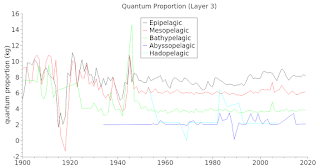Fig. 7 Layer 3Fig. 8 Layer 4Fig. 9 Layer 5Fig. 10 Layer 6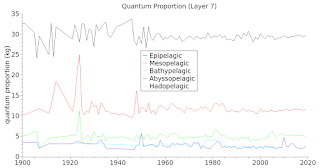Fig. 11 Layer 7Fig. 12 Layer 8
II. A Thing Called 'Que P'

Today I am introducing a 'thing' called 'Qp', which is a symbol for 'quantum proportion'.

Regular readers will remember that I have demonstrated that the Gibbs formulas in the TEOS-10 toolbox can be used to isolate thermodynamic proportion patterns in the ocean depths (e.g. Patterns: Conservative Temperature & Potential Enthalpy - 3, In Search Of Ocean Heat - 8).

The entity 'Qp' is a simple way to track, watch, discover, etc. Conservative Temperature, Potential Enthalpy, and infrared photons in the ocean depths (all at the same time in the same graph lines).

Today's graphs feature WOD "layers" (a Dredd Blog concept, see Fig. 1), Pelagic Depths, quantum proportion mapping in each layer, and finally in all layers combined.

III. What Quantum Proportion Is

Quantum Proportion 'Qp' is the relationship patterns of Conservative Temperature (CT), Potential Enthalpy (hO), and photon quantity (mol/kg) in seawater.

A way of looking at it is that they all have the same DNA, i.e. the same pattern and trend in terms of TEOS-10 calculations.

Remember also the standard TEOS-10 functions for Absolute Salinity (SA) and CT are also related.

To calculate SA one needs in situ salinity (SP), latitude, longitude, pressure (P) (calculated from height (Z); 'height' is a negative value when the in situ measurement location is below the ocean surface):

p = gsw_p_from_z(z,lat)
SA = gsw_SA_from_SP(SP,p,long,lat)

CT is then calculated using the SA value and the in situ seawater temperature (T):

CT = gsw_CT_from_t(SA,t,p)

The potential enthalpy ("Ocean Heat") can then be calculated:

enthalpy = gsw_enthalpy(SA,CT,p)
d = gsw_dynamic_enthalpy(SA,CT,p)

hO = enthalpy - d

The number of infrared photons is determined by dividing hO by the energy per photon  (e=hv).

IV. Calculating Quantum ProportionFig. 13 Layer 9Fig. 14 Layer 10Fig. 15 Layer 11Fig. 16 Layer 12Fig. 17 Layer 13Fig. 18 Layer 14Fig. 19 Layer 15Fig. 20 Layer16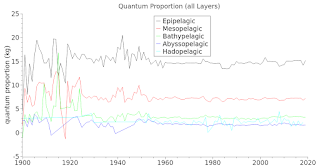Fig. 21 All Layers
Since "over 75% of the ocean is at a depth between 3000 and 6000 m" (Sources of light in the deep ocean) the greatest amount of infrared photons are there [It reminds me of the time a detective was questioning an infamous bank robber: "Why do you rob banks?" the detective asked of the bank robber.

The bank robber quickly replied "Because that is where the money is!"
The Ghost Plumes - 9].

Fig. 3 shows that we can get an indication of how many quanta there are using the 'Qp' formula:

Qp = (CT+hO+photon mols)÷3

I use Qp instead of CT alone, even though CT produces the same proportion pattern.

When simply fishing for a trend or the general pattern CT does the trick, but Qp adds a feel for the magnitude of the ocean heat trend.

The graphs at Fig. 4 thru Fig. 20 will give you a feel for that.

They show the quantum proportion in a kg of seawater at Pelagic Depths (epipelagic, mesopelagic, bathypelagic, abyssopelagic, and hadopelagic).

In other words, from the surface to the bottom of the ocean areas graphed.

If one is looking for an area where tidewater glaciers might be melting at a particular depth, this allows for a quick scan of the ocean heat content available, making it easier to do that.

V. Quantum Exploration

The value of that kind of exploration can be seen in the example of recent Thwaites Glacier research where "A team of scientists has observed, for the first time, the presence of warm water at a vital point underneath a glacier in Antarctica--an alarming discovery that points to the cause behind the gradual melting of this ice shelf while also raising concerns about sea-level rise around the globe.

Warm waters in this part of the world, as remote as they may seem, should serve as a warning to all of us about the potential dire changes to the planet brought about by climate change," explains David Holland, director of New York University's Environmental Fluid Dynamics Laboratory and NYU Abu Dhabi's Center for Global Sea Level Change, which conducted the research. "If these waters are causing glacier melt in Antarctica, resulting changes in sea level would be felt in more inhabited parts of the world.

The recorded warm waters--more than two degrees above freezing--flow beneath the Thwaites Glacier, which is part of the Western Antarctic Ice Sheet. The discovery was made at the glacier's grounding zone--the place at which the ice transitions between resting fully on bedrock and floating on the ocean as an ice shelf and which is key to the overall rate of retreat of a glacier" (Antarctica Thwaites, emphasis added).

The conditions they found were expected, in the sense that TEOS-10 had previously been used here on Dredd Blog to indicate what they actually found (Antarctica 2.0 - 9).

Especially in the sense that some of the researchers emphasized that there was turbulence in addition to seawater conditions that would melt the glacier.

This comports with the plumes (turbulence) that are expected in the calculations of infrared photon induced plumes (The Ghost Plumes, 2, 3, 4, 5, 6, 7, 8, 9).

You might notice that the graph at  Fig. 21 indicates that the deepest ocean depth (Hadopelagic) is warming to the point of becoming warmer than the Abyssopelagic ("hot flows to cold" - laws of thermodynamics).

VI. Photon

I want to make it clear that the photon calculations and QP (Section II) are not part of the TEOS-10 toolbox software.

The "Photon" class is a C++ class I wrote, and is used on Dredd Blog to do photon calculations after I use the TEOS-10 software to calculate enthalpy (see Section III above).

Here is the header file (photon.h) of the C++ class [comments removed]:

#ifndef PHOTON_H
#define PHOTON_H

using namespace std;

const double planckConst = 6.62607004e-34; /** (h) m2 kg / s */
const double lightSpeed_m = 2.99792457999998645e8; /** (c) meters / sec */
const double lightSpeedSq_m = 8.987551787e16; /** (c2) meters / sec sec */
const double avogadroNum = 6.02214154e23; /** Avogadro’s number */
const double nm_to_m_Coeff = 1e-9;

const double wIR = 1.30e-6; /** λm (an infrared wavelength) */
const double vIR = 2.30609583076922e14; /** ν (frequency of wIR) */
const double eIR = 1.52804e-19; /** joules */
const double jMole = 92020.7; /** joules per mole */

struct PHOTON_DATA
{
double nm;
double wavelength;
double frequency;
double e;
double e_mole;
double photonsPerMole;
double moles;

void init()
{
photonsPerMole = wavelength = nm = 0.0;
moles = frequency = e = e_mole = 0.0;
};
};

class Photon
{
public:
Photon();
virtual ~Photon();

PHOTON_DATA &getPhotonData_nm(double nm);
PHOTON_DATA &getPhotonData_wl(double waveLen);
PHOTON_DATA &getPhotonData_f(double frequency);
PHOTON_DATA &getPhotonData_wIR(double joules_kg);

private:
PHOTON_DATA photon_data;
};

#endif

We have a lot of exploring to do but not enough money to do it with.

Researchers doing in situ research first [using WOD in situ measurements], using these techniques, will move us further along in the quest for understanding "the land down under".

We can do TEOS-10 pre-scans that are reliable for developing suspicions about potential glacial melt.

Tons of money can be saved, research advanced, and our knowledge exponentially increased by using quantum science techniques [then focusing on targets indicated to likely be the highest melt areas as indicated by quantum proportion exploration via software logic].

The old pre-quantum-physics based science is just not up to the job.

The next post in this series is here.

Some knew in 1958 and in 1962 ...

#### 1 comment:

1.Antarctica area logs highest temperature on record (link)